American Journal of Electrical Power and Energy Systems
Volume 4, Issue 5, September 2015, Pages: 57-70

Reduction of Total Harmonic Distortion for A Three Phase Fault in a Distribution Network by Using PID, Fuzzy & Hybrid PID-Fuzzy Controller Based DVR

Danish Chaudhary1, Aziz Ahmed1, Anwar Shahzad Siddiqui2

1Dept. of Electrical & Electronics Engg, Alfalah University, Dhauj, Faridabad, India

2Dept. of Electrical Engineering, Jamia Milia Islamia, New Delhi, India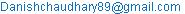(D. Chaudhary)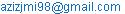(A. Ahmed)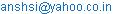(A. S. Siddiqui)

Danish Chaudhary, Aziz Ahmed, Anwar Shahzad Siddiqui. Reduction of Total Harmonic Distortion for A Three Phase Fault in a Distribution Network by Using PID, Fuzzy & Hybrid PID-Fuzzy Controller Based DVR. American Journal of Electrical Power and Energy Systems. Vol. 4, No. 5, 2015, pp. 57-70. doi: 10.11648/j.epes.20150405.11

Abstract: The use of electric energy is, in developed countries around the world, a natural part of life. It is used everywhere for living, work and travelling, at any residence, commercial building, industry and so on. The number of electrical devices connected to the power system, during the century, has increased enormously, with the main increase having been during the last 20 – 25 years. The total power demand has also increased but not at the same rate as the number of devices connected. This is due to more power efficient equipment being used, both for new devices and older replaced devices. There are differences for different voltage levels and of course a large variation among different countries. Due to complexity of power system combined with other factors such as increasing susceptibility of equipment. With electricity demand growing, low power quality is on the rise & becoming notoriously difficult to remedy . Distribution system needs to be protected against voltage sags, dips & swells that adversely affect the reliability & quality of power supply at the utility end. The Dynamic voltage restorer (DVR), which has been utilized in optimized way so as to improve performance, has been put under new technique of sag detection. The applications of Fuzzy logic controller have taken new dimension in various fields. In this paper, the essentials of control scheme with immediate voltage generation to regulate the unbalance voltage phase in three phase system and a tested method to improve the reliability within the distribution system is presented. The 13kV distribution system is having a three phase fault which controlled by non-linear techniques and their performance levels are compared. The capability of DVR is demonstrated using MATLAB/SIMULINK simulation models. This paper emphasizes the importance of DVR application for better power quality, by comparing the mitigated voltage and current THD values among PID, Fuzzy, PID-Fuzzy have been compared on account of the amount of compensation being injected into the system under voltage sag condition for non-linear loads.

Keywords: PID, Fuzzy, PID-Fuzzy, DVR, Total Harmonic Distortion, PWM, Voltage Sags/Swells

1. Introduction

The high quality sinusoidal waveform is produced at power stations. The widespread applications of power electronic based non-linear devices and faults cause deviation from pure sinusoidal waveform. These situations facing electricity customers and suppliers have increased the popularity and development of power quality devices. Users need constant sine wave shape, constant frequency and symmetrical voltage with a constant root mean square (rms) value to continue the production. To satisfy these demands, the disturbances must be eliminated from the system. The typical power quality disturbances are voltage sags, voltage swells, interruptions, phase shifts, harmonics and transients. Power electronic based devices provide protection for industry and commercial customers from power quality problems basically sags, swells & harmonics. These devices are known as custom power devices and they can increase the availability of sensitive loads in the system and supply reliable power. Custom power devices are typically building on the distribution system to provide higher power quality and most economical solution. One of those is DVR used to mitigate voltage sag and well. DVR is a series connected device. It is best custom power device for mitigation impacts of upstream voltage disturbances on sensitive loads .

The economy invested in the distribution system is large enough to take into account the concept of equipment protection against various disturbances that affects the reliability of not only the distribution system but the entire power system incorporating generation & transmission too. The wide acceptance of sophisticated electronic devices at the utility end deteriorates the quality of supply & utility is suffering from its bad effects on large scale. The results of various faults with three phase fault being the most severe among all, starting of induction motor which is most often used due to its rugged construction, switching off large loads and energizing of capacitor banks. This paper attempts to explain the various control strategies providing a reliable solution to the faulted system with the help of DVR (Dynamic Voltage Restorer).

Various control techniques are available to obtain a controlled output voltage, to be injected into the system. They are known as Non-linear techniques like PI controller, PID controller, Fuzzy based controller, by using Artificial Neural Network (ANN) and Hybrid controllers of all above explained controllers. A PID controller with a linear structure offers satisfactory performance over a wide range of operation . The problem encountered by the controller is the setting of PID parameters i.e. the gains (KP, KI, KD). In the influence of varying parameters and operating conditions, the fixed gains of linear controller don’t adapt accordingly to give good dynamic response. To overcome the problems faced by a non-linear technique is an effective solution . The recommended system uses the PID, Fuzzy and Hybrid PID-Fuzzy  controllers to investigate the performance level of various controllers in a regard to increase the capability of the existing system by creating immunity from disturbances. Simulation results of voltage sag condition for a non-linear load are presented.

2. Total Harmonic Distortion

The degree of the voltage distortion varies with the impedance of the electrical power distribution system and the number and type of non-linear loads connected. In order to compare these two distortion levels, it is necessary to quantitatively describe the distortion. Harmonic analysis is used to provide this description. The level of voltage distortion that is acceptable depends on the sensitivity of the equipment installed in the building. In harmonic analysis, any repetitive wave form can be described mathematically as a series of pure sine waves. These sine waves consist of a fundamental frequency and multiples of that frequency, called harmonics.

Total harmonic distortion (THD) is an important figure of merit used to quantify the level of harmonics in voltage or current waveforms . Total harmonic distortion (THD) is often used as a percentage, this single number is calculated by adding the square of each relative harmonic value and taking the square root .

THD =* 100The most detailed method describes the amplitude of each individual harmonic component, either in absolute units (such as volts) or as a percentage of the fundamental component. With this, it is possible to determine the source of harmonic distortion. For example, in a balanced electrical system, the only harmonics that can be generated by a symmetrical three phase load are those that are not multiples of 2 or 3 (the 5th, 7th, 11th, and similar harmonics). If a third harmonic is present in the system, it is likely the result of single phase loads or phase imbalances.

Voltage and current harmonics have undesirable effects on power system operation and power system components. In some instances, interaction between the harmonics and the power system parameters (R–L–C) can cause harmonics to multiply with severe consequences. Voltage harmonics are mostly caused by current harmonics. The voltage provided by the voltage source will be distorted by current harmonics due to source impedance. If the source impedance of the voltage source is small, current harmonics will cause only small voltage harmonics . Harmonics provides a mathematical analysis of distortions to a current or voltage waveform. Based on Fourier series, harmonics can describe any periodic wave as summation of simple sinusoidal waves which are integer multiples of the fundamental frequency.

Power system transients are fast, short-duration events that produce distortions such as notching, ringing, and impulse. The mechanisms by which transient energy is propagated in power lines, transferred to other electrical circuits, and eventually dissipated are different from the factors that affect power frequency disturbances . In electrical engineering, oscillation is an effect caused by a transient response of a circuit or system. It is a momentary event preceding the steady state (electronics) during a sudden change of an event.

3. Dynamic Voltage Restorer

3.1. Introduction

Among the voltage disturbances, voltage sag is most severe that adversely affects the performance of the system. The one such efficient & reliable solution is the DVR.

DVR is a static series compensator that injects voltage in series to the distribution system, regulating the load side voltage. It is connected between the supply and the sensitive load to compensate the line voltage harmonics, reduction of transients in addition to compensation of voltage sags & swells.

3.2. Principle of DVR Operation

The main aim of DVR is to regulate the voltage at the load terminals irrespective of sag, distortion or unbalance in the supply voltage. The basic operating principle is to inject a voltage of required magnitude & frequency to restore the load voltage under voltage sag or distortion. Generally; it employs solid state power electronic switches such as GTO, IGBT or IGCT in the VSI, which can be operated in various pulse width modulation techniques such SPWM(sinusoidal pulse width modulation), MSPWM(multiple sinusoidal pulse width modulation). They inject a set of three phase AC voltage in series & synchronism with the distribution system.

4. Configuration of DVR

The vital components of DVR are the power circuit which injects the desired voltage & control circuit that controls the load voltage of the system within prescribed limits. Its schematic diagram explains the various components as the constituents of DVR as shown in Figure1.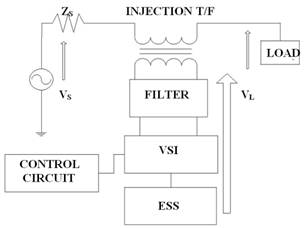Figure 1. Block Diagram of DVR.

4.1. Voltage Source Inverter

It forms the building block of compensating device. It performs the power conversion process from DC to AC. VSI consists of fully controlled semiconductor power switches to form a single phase or three phase topologies. For medium power inverters, IGBT‟s are used and GTO‟s or IGCT‟s due to compact size & fast response for high power inverters are employed. The single phase VSI topology encompasses a low-range power applications and medium to high power applications are covered by the three phase topology . Single phase VSI consists of four semiconductor switches (in 2 legs) to generate the ac output waveform. Three phase VSI is a six step bridge inverter that uses a minimum of six thyristors, where a step means a change in the firing from one thyristor to the next thyristor in proper sequence. For one cycle of 360 degree, each step is of 60 degree for a six step inverter.

4.2. Series Injection Transformer

It provides electrical isolation & voltage boost to the system. In a 3-phase system, either 3 single phase units of isolating transformer or 3-phase isolating transformer can be employed for the purpose of voltage injection. While selecting the injection transformer, the determination of expected maximum output voltage is prime significance, both economically & technically. Prior to the level of the distribution system being compensated by DVR & largest sag to be compensated by VSI at the minimum DC-link voltage decides the turn ratio of the series injection transformer. The effects of higher order harmonics on the transformer are related to the positioning of filtering system, i.e. inverter filtering side system & line side filtering.

4.3. Filter

These are electronic circuits comprising of combination of passive elements; resistors, inductors & capacitors. They perform signal processing functions to remove the unwanted frequency signals to enhance the desired signal output. LC type of filters corrects the harmonic output from VSI to provide compensation in the required phase of the 3 phase system boosted by DVR.

4.4. Energy Storage Unit

The purpose of storage systems is to protect sensitive equipments from shutdown caused by voltage sags or interruptions. They provide necessary energy to the VSI via a dc link for the generation of injected voltages. There are different types of storage systems such as superconducting magnetic energy storage system (SMES), DC batteries, flywheel energy storage system, battery energy storage system (BESS) etc. Capacity of the storage system directly determines the duration of the sag which can be mitigating by the DVR. Among the above mentioned storage systems, Batteries are more common & can be highly effective if high voltage configuration is used. There are different types of battery energy storage technologies such as lead-acid battery, flooded type battery, valve regulated type battery (VLRA), Sodium Sulphur battery (NaS) etc. .

4.5. Control Circuit

Several techniques & control philosophy of the DVR have been implemented for power quality improvement in the distribution system. The DVR is equipped with a control system to mitigate voltage sags/swells. The control of the DVR is very important as it involves the detection of voltage sags (start, end & depth of voltage sag) by appropriate detection algorithm . The control strategy can depend on the type of load connected. Its main purpose is to maintain constant voltage magnitude at the point where the sensitive load is connected under system disturbances. Three basic control strategies of DVR can be stated as:

4.6. Pre-Sag Compensation Method

In this method, both magnitude & phase angle are to be compensated. The supply voltage is continuously tracked & load voltage is compensated to the pre-sag condition by injecting voltage equal to the difference of voltage under pre-sag & sag condition as in Fig 2. Though, it gives a nearly undisturbed load voltage but suffers a drawback of exhausting the rating of the DVR.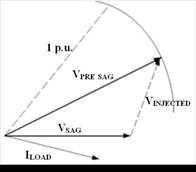Figure 2. Pre-Sag Compensation Method.

4.7. In Phase Compensation Method

In this method, when the source voltage drops due to sagging condition, the VSI injects a voltage called missing voltage based on the drop of voltage magnitude as in fig 3. The generated Voltage of the DVR is always in phase with the measured supply voltage regardless of the load current and the pre-sag voltage.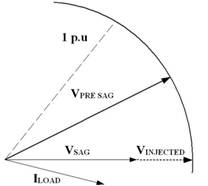Figure 3. In-Phase Compensation Method.

4.8. Reactive Power Compensation

This is also known as the minimum energy injection, which depends on maximizing the active power supplied by the network (keeping the apparent power constant and decreasing the network reactive power) by minimizing the active power supplied by the compensator (increasing the reactive power supplied by the compensator). In this injection method the injected voltage is in quadrature with load current.Figure 4. Reactive Power Compensation.

5. Control Philosophies of DVR

5.1. Introduction

Voltage sags are one of the most severe power quality problems & DVR is an effective solution to mitigate it. The purpose of control scheme is to control the system output by generating an appropriate control signal prior to the unbalanced condition prevailing in the system. It generates the signals to enable the VSI (voltage source inverter) by providing proper firing sequence to the circuit. In this work, different control strategies for dynamic voltage restorer are investigated with emphasis on voltage sag compensation. Three promising control methods to compensate voltage sags are tested & compared with simulation of DVR on 13kV system. The comparison of the performance of three control strategies is made on basis of voltage waveforms & its frequency spectrum analysis. Their performance level is presented in the decreasing order of their compensation capability & better performance in mitigating voltage sag over a broader range for different faults. Three control philosophies have been used namely, PID, Fuzzy & PID-Fuzzy. These are discussed as below:

5.2. Proprotional-Integral-Derivative (PID) Controller Based DVRFigure 5. Control Strategy of PID Controller.

PID is a feedback controller that uses the weighted sum of error & its integral value to perform the control operation. The proportional response can be adjusted by multiplying the error by constant KP, called proportional gain. The contribution from integral term is proportional to both the magnitude of error and duration of error. The error is first multiplied by the integral gain, KI and then was integrated to give an accumulated offset that have been corrected previously . Derivative term is also proportional to both error magnitude and duration of error. The error is first multiplied by differential gain KD and then was differentiated to give an accumulate offset that have been corrected. This derivative gain increases the speed of error correction while integral gain removes the error completely. The input to the PID controller is difference between the reference value & error value of voltage. As per the comparison of reference value & error value of voltage, linear PID adjusts its proportional, integral and differential gains KP, KI and KD in order to reduce the steady state error to zero for a step input as shown in Figure5. It is widely used due to simple control structure but suffers a disadvantage of fixed gains i.e. it cannot adapt itself to the varying parameters & conditions of the system.

5.3. Fuzzy Controller Based DVR

The drawback suffered by PID controller is overcome by Fuzzy. In comparison to the linear PID controller, this is a non-linear controller that can provide satisfactory performance under the influence of changing system parameters & operating conditions  . The function fuzzy controller is very useful as relieves the system from exact & cumbersome mathematical modeling & calculations. The performance of fuzzy controller is well established for improvements in both transient & steady state . The fuzzy controller comprises of four main functional modules namely; Knowledge base, Fuzzification, Inference mechanism & Defuzzification as in figure 6.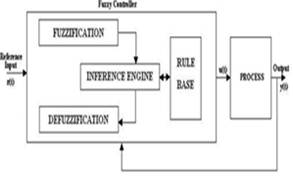Figure 6. Schematic Diagram of Fuzzy Logic.

5.4. Knowledge Base

It consists of data base & rule base that maps all the input & output with certain degree of uncertainty in process parameters & external disturbances to obtain good dynamic response. Data base scales the input-output variables in the form of membership functions that defines it in a range appropriate to provide information to the fuzzy rule-based system & output variables or control actions to the system under observation. Fuzzy rule-based system utilizes a collection of fuzzy conditional statements derived from a knowledge base to approximate and construct the control surface.

5.5. Fuzzification

It is the process of defining a crisp data or digital data operating on discrete values of either 0 or 1 in terms of logical variables that take on continuous values between 0 and 1 i.e. fuzzy set. Fuzzy set maps the input-output variables into membership functions & truth values as in figure 7 & figure 8.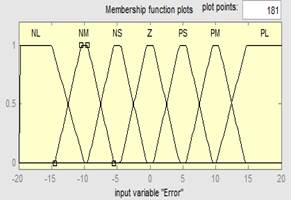Figure 7. Input Membership Function of "Error".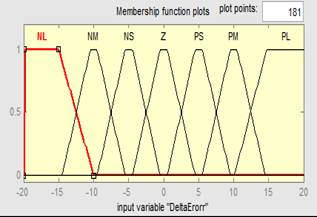Figure 8. Input Membership Function of "Change in Error".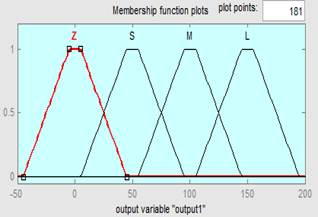Figure 9. Output Membership Function.

5.6. Inference Mechanism

It is referred to as approximate reasoning that uses knowledge to conduct deductive inference of IF-THEN rules. This mechanism encodes knowledge about a system in statements form of linguistic IF-THEN propositions with antecedents & consequents.

Ÿ Defuzzification

It is a conversion process of fuzzy quantity to a precise quantity and is reverse process of fuzzification. A logical union of two or more membership functions in the universe of discourse requires a crisp decision with approximate solution for the output of fuzzy which is uncertain in nature to be a single scalar quantity

The FLC controller of the tested system exploits the Mamdani type of inference method. It defuzzifies the crisp input-output variables into fuzzy trapezoidal membership function and reverse process of Defuzzification is based upon the Centroid method. The controller core is the fuzzy control rules as shown in table I. which are mainly obtained from intuitive feeling and experience .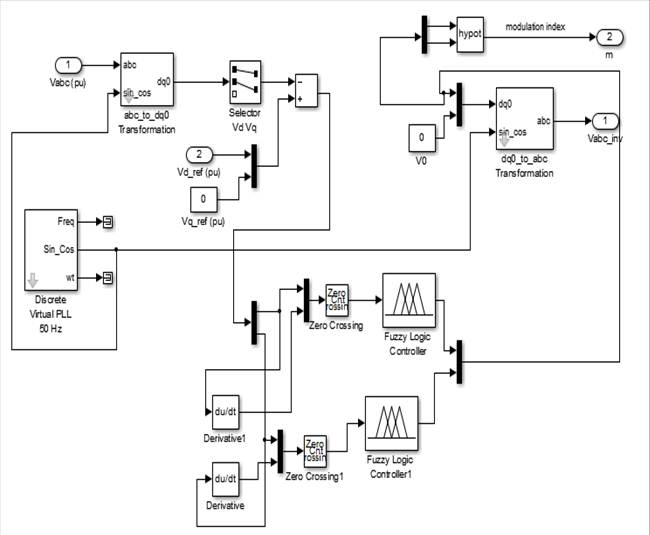Figure 10. Control Strategy of Fuzzy Controller.

Table 1. Fuzzy Rule Based System.

 "e" "ce" NL NM NS Z PS PM PL NL L L L M Z S Z NM L L M Z Z Z S NS L M S Z Z S S Z M S S Z S S M PS S S Z Z S M L PM S Z Z Z M L L PL Z S Z M L L L

5.7. Hybrid PID-Fuzzy Based DVR

The hybrid PID-Fuzzy control scheme uses fuzzy as adjustor to adjust the parameters of proportional gain KP, integral gain KI and derivative gain KD based on the error e and the change of error e . PID-Fuzzy based Controller has been designed by taking inputs as error which is difference between measured voltage and reference voltage of DVR for voltage regulator and its derivative while ΔKP, ΔKI and ΔKD as output for voltage regulator where KP, KI and KD are proportional gain, integral gain and derivative gain respectively  as shown in Figure11.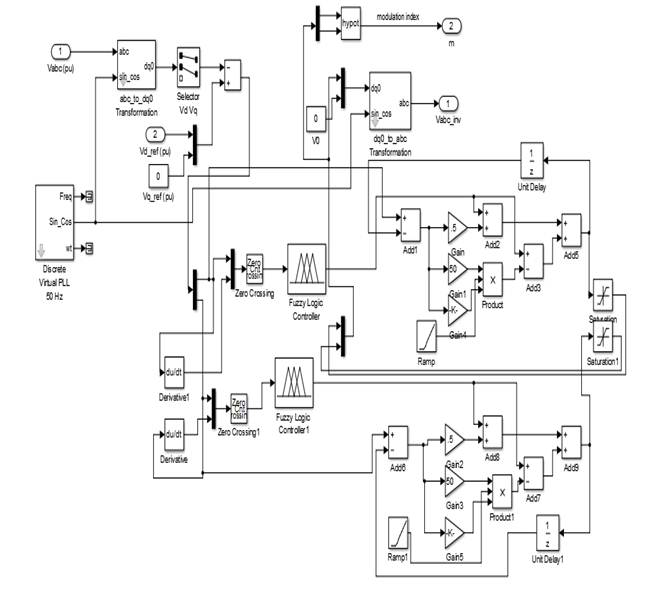Figure 11. Control Strategy of PID FUZZY Controller.

6. Simulation Test Models

6.1. Introduction

In the SIMULINK test model, two feeders are drawn from the same supply using 3- winding transformer. One of the feeders is compensated using DVR while the other uncompensated. The parameters for the whole system model are explained in Table 2. These are further connected to identical loads so that their performances are fairly compared. The controllers PID, Fuzzy, hybrid PID-Fuzzy are employed step by step in the compensated feeder to compare their performances. The single line test diagram is shown in Figure12.

Table 2. System Parameters.

 Sr.No. System Quantities Standards 1. Source 3-Phase,13kV,50Hz 2. Inverter Parameters IGBT based,3arms,6 Pulse, Carrier frequency -1080Hz Sample time=50μsec 3. PID Controller KP=0.5,KI=50, KD=100, sample time=50μsec 4. RL Load Active power = 1 KW Reactive power = 400 VAR 5. Three Winding Transformer Υ/Δ/Δ 13/115/115kV 6. TwoWinding Transformer Δ/Υ 115/11 kV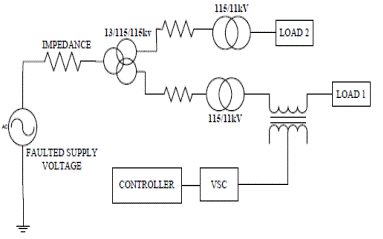Figure 12. Single Line Diagram of Test Circuit.

6.2. Simulink Model of the Test System with PID Controller

In the SIMULINK model, the PID controller based DVR is investigated during a three phase fault to remove the voltage sag arises in output waveform due to the fault as shown in Figure13. In the simulation results, we study the output waveform to be uniformly sinusoidal after voltage magnification and the amount of harmonics reduced from during fault to post fault condition by using PID controller based DVR.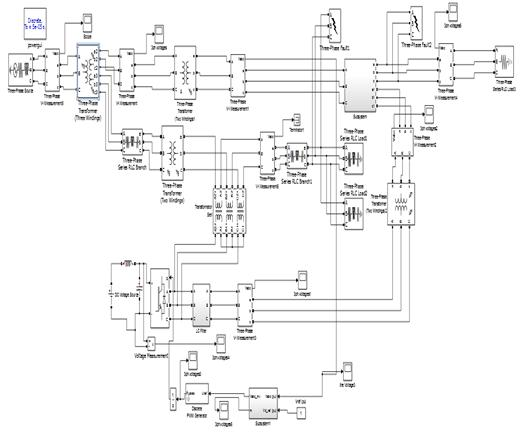Figure 13. Simulink Model of PID Controller Based DVR.

6.3. Simulink Model of Test System with Fuzzy Controller

In this case, fuzzy logic controller is employed to compensate the uncompensated system shown in Figure14. It overcomes the disadvantage suffered with linear PID controller by providing better compensation and reducing the THD level of uncompensated system. In here simulation results, we comparatively study its output waveform with previous result of PID controller based DVR, and also the variation in THD for both PID and Fuzzy controller based DVR.Figure 14. Simulink Model of FUZZY Based DVR.Figure 15. Simulink Model of HYBRID PID FUZZY Controller Based DVR.

6.4. Simulink Model of Test System with Hybrid PID-Fuzzy Controller

In this case, hybrid PID-Fuzzy control scheme is employed and tested for a three phase fault in a distribution network. This hybrid controller adjusts the proportional, integral gains and derivative gain KP, KI and KD of PID using the trapezoidal membership function and the rule base system for regulating the voltage of the system as shown in Figure11. The complete simulation test model for hybrid PID-Fuzzy controller based DVR is shown in Figure15.

7. Result

After simulating the test model during a three phase fault in a distribution network we get the waveform during fault as shown in Figure16. which clearly shows the voltage sag during a three phase fault i.e. from time 0.04 sec to 0.10 sec after which the fault is removed leaving behind the higher order harmonics which is shown in the Figure16. The frequency spectrum analysis shows the percentage of Total Harmonic Distortion during fault condition is shown in Figure17. The value for THD during a three phase fault for a given test system is 23.67%.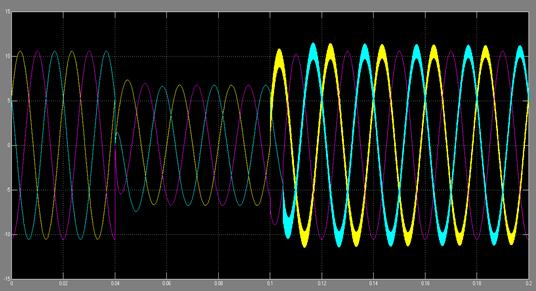Figure 16. Load Voltage Waveform During Three Phase Fault.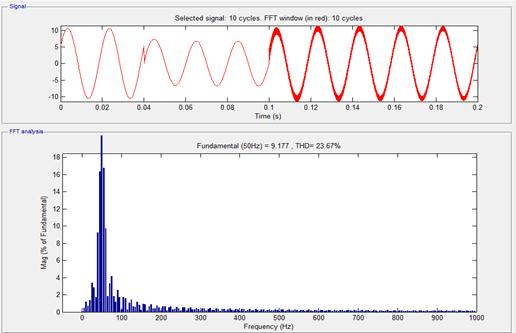Figure 17. FFT Analysis of when Uncompensated During Three Phase Fault .

Now after simulating all the three controlling techniques i.e. PID, Fuzzy and Hybrid PID-Fuzzy controller based DVR respectively for the removal of a three phase fault, we get the following results:

Simulation Result for PID Controller Based DVR

The resultant output waveform after mitigation of fault in the network is shown in the Figure18. In the output waveform we have seen that the voltage sag is removed but a slightly distorted waveform will remain after fault clearing time as in Figure18.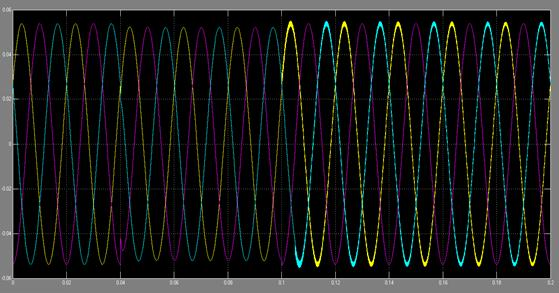Figure 18. Output Voltage Waveform when Compensated Using PID Controller Based DVR.

Figure19.shows the frequency spectrum analysis showing the THD at a fundamental voltage level. Now after clearing the fault, PID controller reduces the THD from 23.67% (during fault) to 3.09% (by using PID compensator).Figure 19. Load Frequency Spectrum when Compensated Using PID Controller Based DVR.

Simulation Result for Fuzzy Controller Based DVR

By using FLC, the output waveform will remain same only the distortion which is coming after the fault clearing time is minimized to further extent as shown in Figure20.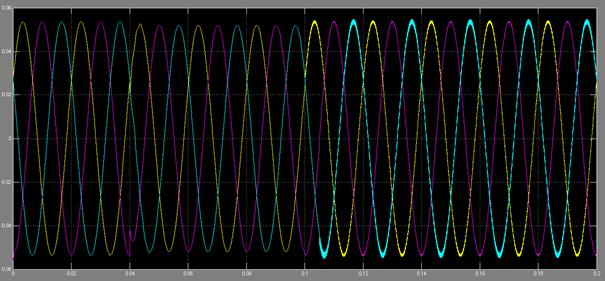Figure 20. Load Voltage Waveform when Compensated Using FUZZY Controller Based DVR

Figure21. shows the FFT analysis for Fuzzy controller based DVR for compensation of a three phase fault. From the graph we can see that the THD is further more reduced from 23.67% to 2.58% while the percentage of THD was 3.09% for PID compensated system.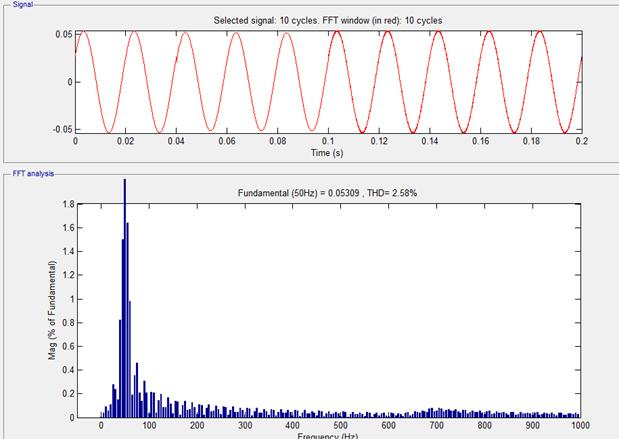Figure 21. Load Frequency Spectrum when Compensated Using FUZZY Controller Based DVR.

Simulation Result for Hybrid PID-Fuzzy Controller Based DVR

As it is the combination of both the above explained compensation techniques, so the results for this controller are supposed to be good and the results are really much up to our expectations as shown in the Figure22. The harmonics in the output waveform are further reduced to certain level.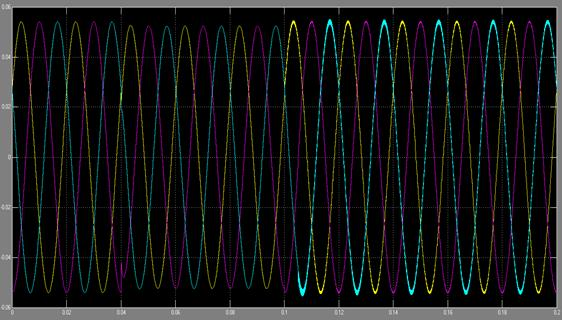Figure 22. Load Voltage Waveform When Compensated using PID FUZZY Controller Based DVR.

The values for the reduced THD are shown in load frequency spectrum for hybrid PID-Fuzzy controller based DVR in Figure23. The percentage for THD is now reduced to a level of 2.42% which is really less than 3.09% of PID controller and as well as 2.58% of Fuzzy controller.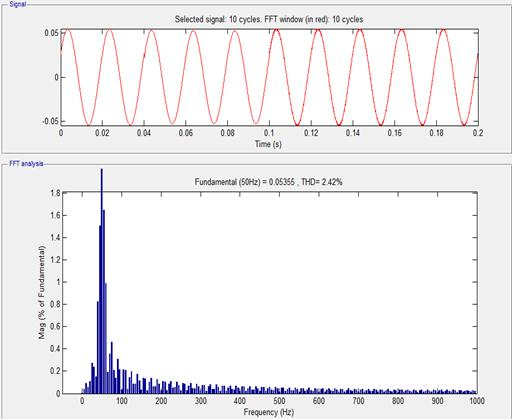Figure 23. Load Frequency Spectrum When Compensated using PID FUZZY Controller Based DVR.

As given in the Table 3, all the three control strategies namely, PID controller based DVR, Fuzzy controller based DVR and hybrid PID-Fuzzy based DVR give the respective values as shown in the Table no. 3 during a three phase fault in the system. In which hybrid PID-Fuzzy gives better compensation by reducing the THD level of uncompensated system from 23.67% to a much reduced value of 2.42%% as compared to 2.58% with Fuzzy controller and 3.09% with PID controller.

Table 3. THD Values for Different Control Strategies

 Controllers Load voltage (50Hz) THD (%) PID 0.05333 3.09% FUZZY 0.05359 2.58% PID- FUZZY 0.05355 2.42%

8. Conclusions

In this work, the various control strategies are employed and tested for 13kV distribution system. The PID controller based DVR, fuzzy controller based DVR and PID-Fuzzy controller based DVR’s are connected step by step in the compensated feeder to compare their performances. The effectiveness of different control techniques based DVRs for three phase fault have been investigated. As seen from the load voltage waveform and frequency spectrum of uncompensated system, the THD level is reduced effectively from 23.67% to a much less value of 3.09% in case of PID controller. Load voltage waveforms and frequency spectrum of Fuzzy control scheme for a three phase fault depicts that the harmonics are effectively reduced to a less value as compared to 2.58% with Fuzzy controller and 2.42% with Hybrid PID-Fuzzy controller. Simulation results indicate that Hybrid PID-Fuzzy compensation techniques provide better compensation to the system as compared to the linear PID technique or Fuzzy controller based DVR connected to the feeder during three phase fault.

References

1. Rosli Omar, N.A.Rahim, Marizan Sulaiman, "Dynamic Voltage Restorer Application for Power Quality Improvement in Electrical Distribution System: An Overview", Australian Journal of Basic and Applied Sciences, vol.5, pp.379-396, December 2011.
2. R. H.Salimin and M. S. A.Rahim, "Simulation Analysis of DVR Performance for Voltage Sag Mitigation", IEEE 5th International Conference iineering and Optimization, pp.261-266, June 2011.
3. Ravilla Madhusudanl, G. Ramamohan Rao"Modeling and Simulation of a Dynamic VoltageRestorer (DVR) for Power Quality Problems Voltage Sags and Swells", IEEE- International Conference On Advances In Engineering, Science And Management (ICAESM -2012) March 30, 31,2012 442.
4. Jurado, Francisco, Valverde, M, "Fuzzy Logic Control of a Dynamic Voltage Restorer", IEEE International Symposium on Industrial Electronics", vol. 2, pp.1047-1052, May 2004.
5. A.Luo, C.Tang, Z.Shuai, J.Tang, X.Y. Xu, D.Chen, "Fuzzy-PID Based Direct-Output- Voltage Control Strategy for the Statcom Used in Utility Distribution systems", IEEE Transactions on Industrial Electronics, vol.56, pp.2401-2411, July 2009.
6. S.Chauhan, V. Chopra, S.Singh, "Power System Transient Stability Improvement Using Fuzzy-PID Based STATCOM Controller", 2nd International Conference on Power, Control and Embedded Systems, pp.1-6, December 2012.
7. M.Srivatsa, S. Srinivasa Rao "THD analysis with custom power devices at distorted load conditions: Implementation of UPQC using PI-Resonant controller" International Journal of Innovative Research in Science, Engineering and Technology Volume 3, Special Issue 1, February 2014 International Conference on Engineering Technology and Science-(ICETS’14)
8. RAMANPREET KAUR, PARAG NIJHAWAN"Comparative Analysis Of The Pi, Fuzzy And Hybrid Pi-Fuzzy Controllers Based DVR", Thapar University.
9. Harkesh Pathak, Mohd. Iliyas "Power Quality Improvement Of Distribution Networks Using Dynamic Voltage Restorer" Department of Electrical Enginering, AFSET.
10. U.V Krishnan, M.Ramasamy, "An Enhancement Method for the Compensation of Voltage Sag/Swell and Harmonics by Dynamic Voltage Restorer", International Journal of Modern Engineering Research, vol.2, pp.475-478, March-April 2012.
11. H.P.Tiwari and S.K.Gupta, "Dynamic Voltage Restorer Against Voltage Sag", International Journal of Innovation, Management and Technology, vol.1, pp.232-237, August 2010.  P.C Loh, D.M Vilathgamuwa, S.K Tang, H.L Long, "Multilevel Dynamic Voltage Restorer", International Conference on Power System Technology, vol.2, pp.1673-1678, November 2004.

 Contents 1. 2. 3. 3.1. 3.2. 4. 4.1. 4.2. 4.3. 4.4. 4.5. 4.6. 4.7. 4.8. 5. 5.1. 5.2. 5.3. 5.4. 5.5. 5.6. 5.7. 6. 6.1. 6.2. 6.3. 6.4. 7. 8.
Article ToolsAbstractPDF(800K)